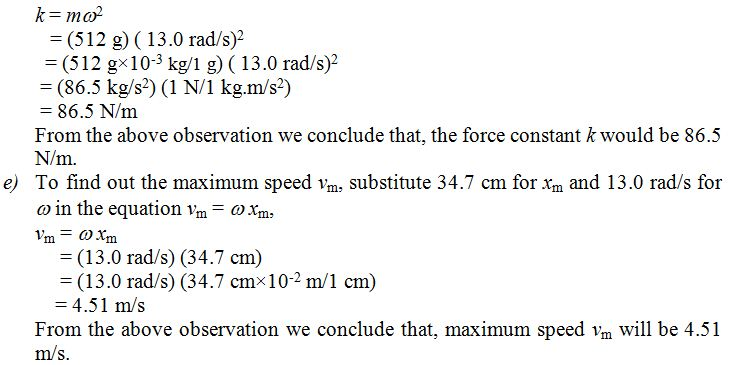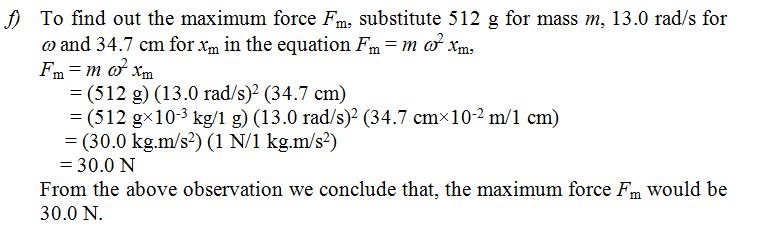×#### Thank you for registering.

One of our academic counsellors will contact you within 1 working day.

Click to Chat

1800-1023-196

+91-120-4616500

CART 0

• 0

MY CART (5)

Use Coupon: CART20 and get 20% off on all online Study Material

ITEM
DETAILS
MRP
DISCOUNT
FINAL PRICE
Total Price: Rs.

There are no items in this cart.
Continue Shopping
```
An oscillator consists of a block of mass 512 g connected to a spring. When set into oscillation with amplitude 34.7 cm, it is observed to repeat its motion every 0.484 s. Find (a) the period, (b) the frequency, (c) the angular frequency, (d) the force constant, (e) the maximum speed, and (f) the maximum force exerted on the block.

```
5 years ago396 Points
```							(a) Since the motion is repeated in every 0.484 s, therefore the period of oscillation T will be,T = 0.484 s(b) To find out the frequency f of oscillation, substitute T = 0.484 s in the equation f = 1/T,f = 1/T= 1/0.484 s= (2.07/s) (s / 1/Hz)= 2.07 HzFrom the above observation we conclude that, frequency of the oscillation would be 2.07 Hz.(c) To find out the angular frequency w of the oscillation, substitute 2.07 Hz for f in the equation w = 2πf,w = 2πf= 2×3.14×2.07 Hz= 2×3.14×(2.07 Hz× (1/1 sec)/1 Hz)= 2×3.14×2.07/s= 13.0 rad/sFrom the above observation we conclude that, angular frequency w of the oscillation would be 13.0 rad/s.(d) To find out the force constant k, substitute 512 g for m and 13.0 rad/s for w in the euation k = mw2```
5 years ago
```2(a) Since the motion is repeated in every 0.484 s, therefore the period of oscillation T will be,T = 0.484 s(b) To find out the frequency f of oscillation, substitute T = 0.484 s in the equation f = 1/T,f = 1/T= 1/0.484 s= (2.07/s) (s / 1/Hz)= 2.07 HzFrom the above observation we conclude that, frequency of the oscillation would be 2.07 Hz.(c) To find out the angular frequency w of the oscillation, substitute 2.07 Hz for f in the equation w = 2pf,w = 2pf= 2×3.14×2.07 Hz= 2×3.14×(2.07 Hz× (1/1 sec)/1 Hz)= 2×3.14×2.07/s= 13.0 rad/sFrom the above observation we conclude that, angular frequency w of the oscillation would be 13.0 rad/s.(d) To find out the force constant k, substitute 512 g for m and 13.0 rad/s for w in the euation k = mw
```
5 years ago
```							TRY AGAIN
```
5 years ago
Think You Can Provide A Better Answer ?

## Other Related Questions on Wave Motion

View all Questions »### Course Features

• 101 Video Lectures
• Revision Notes
• Previous Year Papers
• Mind Map
• Study Planner
• NCERT Solutions
• Discussion Forum
• Test paper with Video Solution### Course Features

• 110 Video Lectures
• Revision Notes
• Test paper with Video Solution
• Mind Map
• Study Planner
• NCERT Solutions
• Discussion Forum
• Previous Year Exam Questions